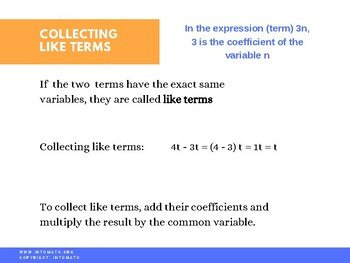CA$2.00 What is the difference between expressions and equations? What does it mean “to solve an equation”? The answers to the above questions are provided in this lesson. An equation is an equality of two quantities and it always contains an equal sign. To solve an equation means to determine the value of the unknown(s) that makes the equation true, so that when the unknown is being substituted with the value in the equation, the left side is equal the right side. An expression does not have an equal sign, but it may contain variables that represent a certain quantity or relationship. To simplify an expression means to complete operations withing the expression that would bring it to its simplest form. In order to do that collecting like terms ins required. Like terms are terms that have different coefficients but identical variables with the same exponent.Grade 7 Workbook (22 pages with answers) CA$4.00

You are going to learn how to solve a linear equation by completing the reverse BEDMAS and performing opposite operations. For example, an equation 2x + 3 = 9 could be solved by first subtracting 3 from both sides of the equation and then dividing both sides of the equation by 2. Thus, x = 3. It is important to make sure that the same operation is done to both sides of the equation to keep the equation balanced.

Collecting like terms is often necessary on one or both sides of the equation.

Doing the “left side, right side check” is an important component of solving equations, as it would help you determine whether the solution is correct. This lesson will teach you how to do it.